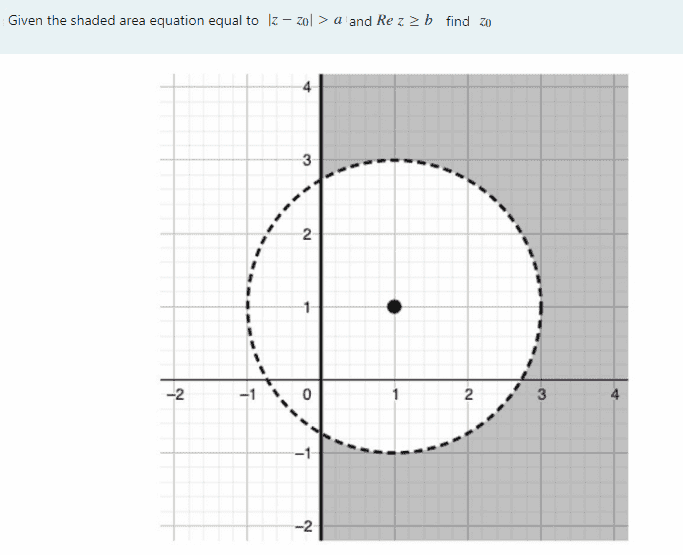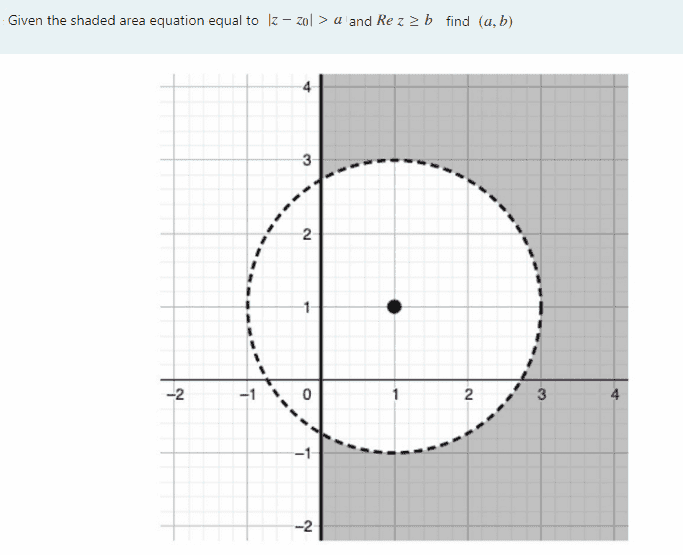# Complex number equation graph problem

• MHB
• jaychay
In summary, you are looking for the equation for Zo and the coordinate (a,b) in a coordinate system. You are given that Zo is equal to (1,1) and that (a,b) is located in the shaded area in the opening post.f

#### jaychayGiven (a,b) is the coordinate just like (x,y). Find equation Zo and coordinate (a,b) ?

Last edited:
Is the answer $z_o = (1,1)$ or simply $1+i$ and $(a, b)=(2, 1.8)$ ? Not sure about b though

Is the answer $z_o = (1,1)$ or simply $1+i$ and $(a, b)=(2, 1.8)$ ? Not sure about b though
Can you tell me where does (a,b) = ( 2,1.8 ) come from ?

first of all $(a, b)$ are not co-ordinates... a represents the radius of circle centered at $z_o$ .
For b see the graph... from the circle we can say z must be outside the circle then it's real part should also be outside it(see the minimum value of Re(z) so that it is outside the circle)

I am really struggle with question 2 on how to find (a,b) I am not sure that b = 1.8 is correct or not

I am really struggle with question 2 on how to find (a,b) I am not sure that b = 1.8 is correct or not
We are given the inequality $\operatorname{Re}z\ge b$ and in the graph we can see that the shaded area is to the right of the imaginary axis.
Therefore $b=0$.

We are given the inequality $\operatorname{Re}z\ge b$ and in the graph we can see that the shaded area is to the right of the imaginary axis.
Therefore $b=0$.
So ( a,b ) is equal to ( 2,0 ) right ?

So ( a,b ) is equal to ( 2,0 ) right ?
Yes.

We are given the inequality $\operatorname{Re}z\ge b$ and in the graph we can see that the shaded area is to the right of the imaginary axis.
Therefore $b=0$.
Sir you are saying that Re(z) = 0 that means z =0 + i y means z will lie on imazinary axis but from the graph z lies in 1st and 4th quadrants except inside the circle. How is this possible?

Yes.
On question one Zo is equal to (1,1) right ?

Sir you are saying that Re(z) = 0 that means z =0 + i y means z will lie on imazinary axis but from the graph z lies in 1st and 4th quadrants except inside the circle. How is this possible?
We don't have $\operatorname{Re}(z)=0$ for the shaded area. Instead we have $\operatorname{Re}(z)\ge 0$.

[DESMOS]{"version":7,"graph":{"viewport":{"xmin":-10,"ymin":-6.756756756756757,"xmax":10,"ymax":6.756756756756757}},"randomSeed":"fb6b112a792f74beca093fcec90d2a8f","expressions":{"list":[{"type":"expression","id":"1","color":"#c74440","latex":"x\\ge0"},{"type":"expression","id":"3","color":"#388c46"}]}}[/DESMOS]

The combination of $|z-(1+i)|>2$ and $\operatorname{Re} z\ge 0$ shows up as:

[DESMOS]{"version":7,"graph":{"viewport":{"xmin":-10,"ymin":-6.756756756756757,"xmax":10,"ymax":6.756756756756757}},"randomSeed":"5dafa6730d6be2f3a851403cb787a49a","expressions":{"list":[{"type":"expression","id":"1","color":"#c74440","latex":"\\left(x-1\\right)^{2}+\\left(y-1\\right)^{2}>2^{2}\\left\\{x\\ge0\\right\\}"},{"type":"expression","id":"2","color":"#2d70b3"},{"type":"expression","id":"3","color":"#388c46"}]}}[/DESMOS]

As we can see, this matches the shaded area in the opening post.

On question one Zo is equal to (1,1) right ?
I'd make it $z_0=1+i$, since $z_0$ is an imaginary number.
To be fair, that is equivalent to $(1,1)$, which is the cartesian representation of the same imaginary number.